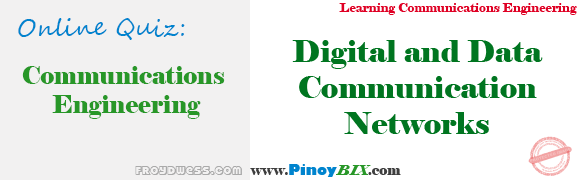# Practice Quiz in Digital and Data Communication Networks Part 1

(Last Updated On: December 20, 2017)This is the Online Practice Quiz in Digital and Data Communication Networks Part 1 as one of the Communications Engineering topic. In Preparation for the ECE Board Exam make sure to expose yourself and familiarize in each and every questions compiled here taken from various sources including but not limited to past Board Examination Questions in Electronic System and Technologies, Communications Books, Journals and other Communications References.

### Start Part I of the Online Practice Quiz

#### Quiz in Digital and Data Communication Networks

Question 1. The EIA standard specified in the EIA-232 standard is ______ volts.

A. Greater than -15

B. Less than -15

C. Between -3 and -15

D. Between 3 and 15

Question 2. In trellis coding. The number of the data bits is _____ the number of transmitted bits.

A. Equal to

B. Less than

C. More than

D. Double that of

Question 3. For a quaternary phase shift keying (QPSK) modulation, data with a carrier frequency of 70 MHz, and input bit rate of 10 Mbps, determine the minimum Nyquist bandwidth.

A. 10 MHz

B. 5 MHz

C. 20 MHz

D. 40 MHz

Question 4. A coherent binary phase shift keyed (BPSK) transmitter operates at a bit rate of Mbps with a carrier to noise ratio C/N of 8.8 dB. Find Eb/No.

A. 8.8 dB

B. 16.16 dB

C. 21.81 dB

D. 18.8 dB

Question 5. For sample rate of 30 kHz in a PCM system, determine the maximum analog input frequency.

A. 30 kHz

B. 15 kHz

C. 60 kHz

D. 45 kHz

Question 6. In QAM, both phase and ________ of a carrier frequency are varied.

A. Amplitude

B. Frequency

C. Bit rate

D. Baud rate

Question 7. The signal between two modems is always

A. Digital

B. Analog

C. PSK

D. QAM

Question 8. A telephone line has a bandwidth of 3.2 kHz and a signal-to-noise ratio of 34 dB. A signal is transmitted down this line using a four-level code. What is the maximum theoretical data rate?

A. 12.8 kbps

B. 6.4 kbps

C. 36.144 kbps

D. 18.072 kbps

Question 9. Data are sent over pin ________ of the EIA-RS-232 interface.

A. 2

B. 3

C. 4

D. All of these

Question 10. An asynchronous communications system uses ASCII at 9600 bps with eight bits, one start bit, one stop bit and no parity bit. Express the data rate in words per minute. (Assume a word has five characters and one space).

A. 9600 wpm

B. 57600 wpm

C. 160 wpm

D. 11520 wpm

Rate this: